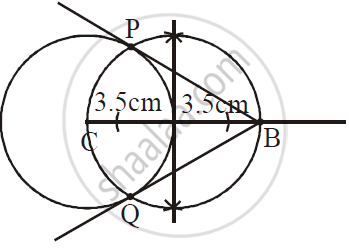SSC (English Medium) Class 10th Board ExamMaharashtra State Board
Share

# Draw the Circle with Centre C and Radius 3.5 Cm. Point B is at a Distance 7 Cm from the Centre. Draw Tangents to the Circle from the Point B. - SSC (English Medium) Class 10th Board Exam - Geometry

ConceptConstruction of Tangent to the Circle from the Point on the Circle

#### Question

Draw the circle with centre C and radius 3.5 cm. Point B is at a distance 7 cm from the centre. Draw tangents to the circle from the point B.

#### Solution1) Take centre C

2) Draw circle of radius 3.5 cm

3) Extend CA till CB where l(CB) = 7cm

4) Take distance more than half in compass and mark two arcs from the ends to line CB.

5) Now draw two more arcs above and below the line keeping the compass on these arcs.

Is there an error in this question or solution?

#### Video TutorialsVIEW ALL 

Solution Draw the Circle with Centre C and Radius 3.5 Cm. Point B is at a Distance 7 Cm from the Centre. Draw Tangents to the Circle from the Point B. Concept: Construction of Tangent to the Circle from the Point on the Circle.
S